Caffe-Code

 - 学习样本语义特征嵌入，使得在语义嵌入空间中，相似样本映射距离更接近，不相似样本映射距离更远.

 - 更好的利用网络训练中 batch 训练的优势，提出将一个 batch 内样本的成对距离向量升级为成对距离矩阵(lifting the vector of pairwise distances within the batch to the matrix of pairwise distances).

 - 问题被转化为了一个 multiclass label 问题.

## 1. Introduction

### 1.1. Contrastive embedding

contrastive training 最小化相同类别的成对样本间的距离，惩罚 negative 成对距离小于边缘参数 ${ \alpha }$ .

$${ J = \frac{1}{m} \sum_{(i, j)}^{m/2} y_{i, j} D_{i, j}^2 + (1-y_{i,j})[\alpha- D_{i, j}]_{+}^2 }$$

m - batch 内图片数，即batchsize.

${ f(\cdot) }$ - 网络输出的特征嵌入.

${ D_{i, j} = ||f(\pmb{x}_i)- f(\pmb{x}_j)||_2 }$

${ y_{i, j} \in {0, 1} }$ - 表示一对样本 ${ (\pmb{x}_i, \pmb{x}_j) }$ 是否是同类样本.

${ [\cdot]_+ }$ - 表示 hinge 函数 ${ max(0, \cdot) }$ 操作.

### 1.2 Triplet embedding

triplet training 寻找一个语义嵌入，使得 $\lbrace (\pmb{x}_a^{(i)}, \pmb{x}_n^{(i)}) \rbrace$ 间的距离大于 $\lbrace (\pmb{x}_a^{(i)}, \pmb{x}_p^{(i)}) \rbrace$ 间的距离加上一个边缘参数 ${ \alpha }$.

$${ J = \frac{3}{2m} \sum_{i}^{m/3} [D_{ia, ip} ^2- D_{ia, in}^2 + \alpha]_{+} }$$

$${ D_{ia, ip} = ||f(\pmb{x}_i^a)- f(\pmb{x}_j^p)|| }$$

$${ D_{ia, ip} = ||f(\pmb{x}_i^a)- f(\pmb{x}_i^n)|| }$$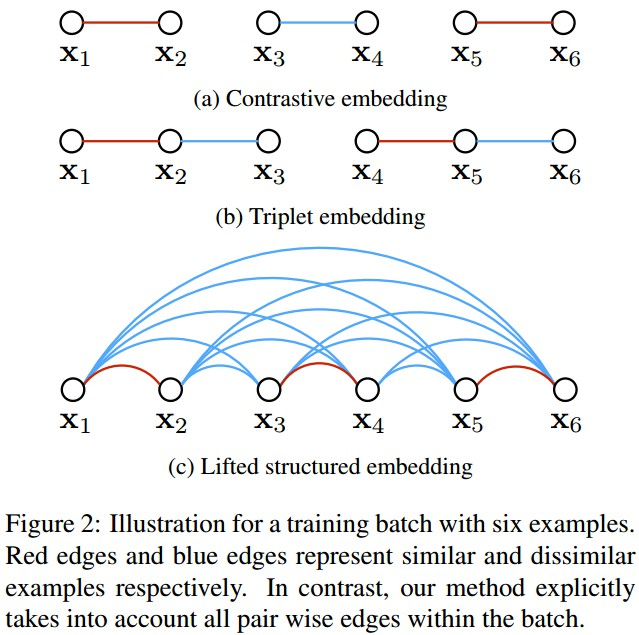Figure 2. 六个样本的 training batch 的不同训练例示. 红色线和蓝色线分别表示相似和不相似的样本. 对比而言，Lifted structured embedding 能够考虑 batch 内所有的成对距离.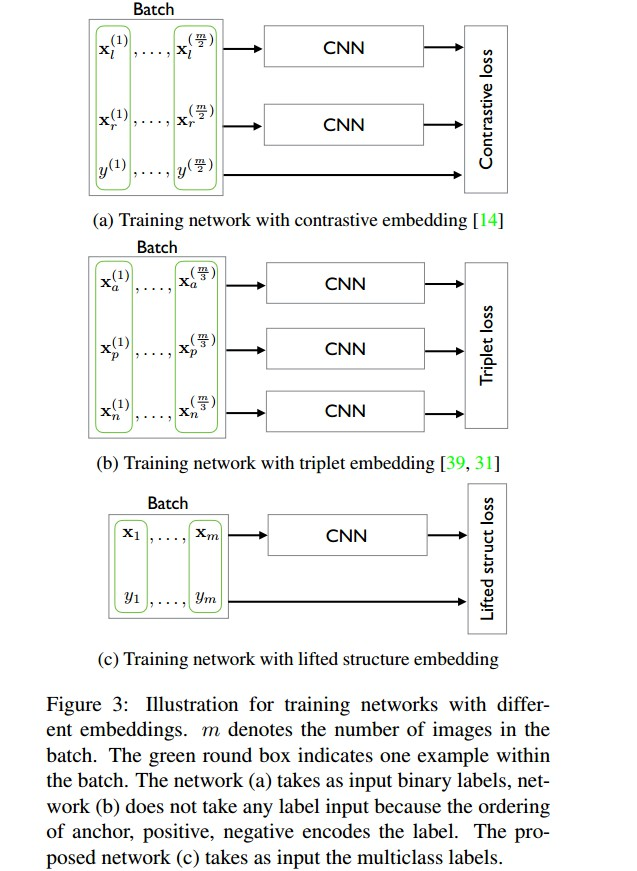Figure 3. 不同嵌入方法的训练网络例示. m 表示 batch 内图片数，即batchsize. 绿色框表示一个 batch 的一个样本.

(a) 网络采用二值labels作为输入；

(b) 网络不需要 label 输入，anchor , positive 和 negetive 的顺序编码了 label 信息；

(c) 网络采用 multiclass label 作为输入.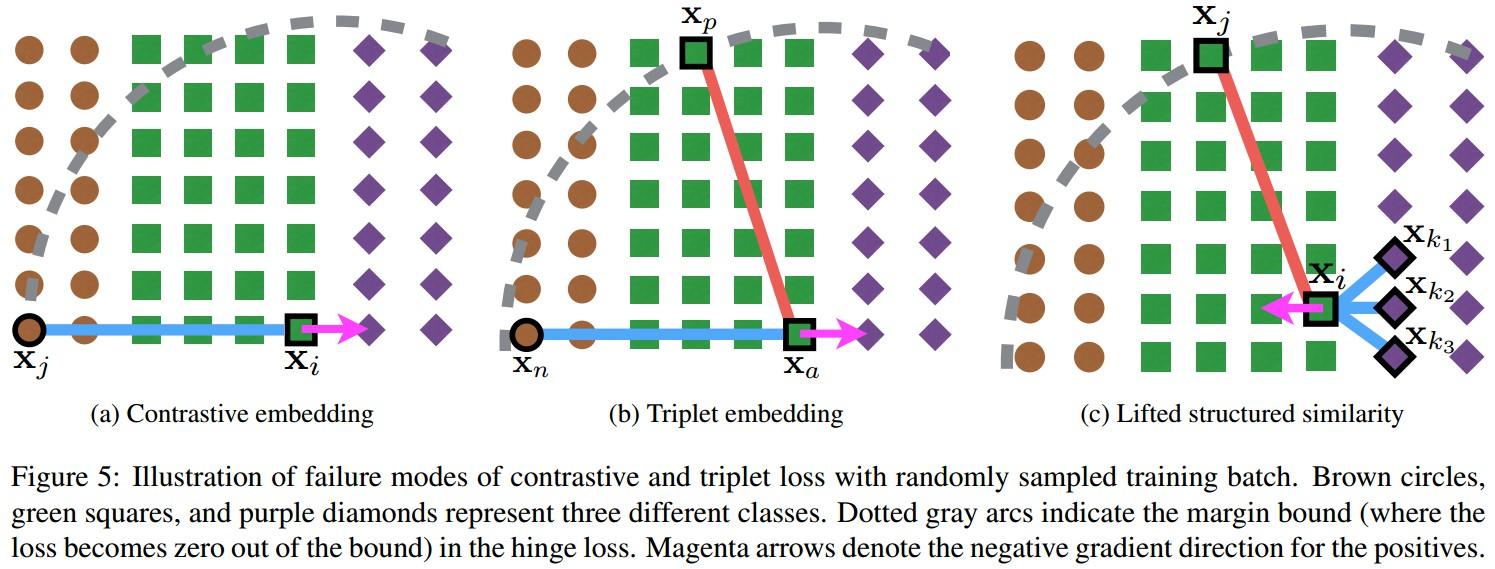Figure5. 随机对 training batch 采样，采用contrastive loss 和 triplet loss 失败的情况. 这里以三类为例，分别对应棕色圆、绿色方块和紫色棱形. 虚线灰色弧线表示在 hinge loss 中的边界(超出边界后，loss变为0). 品红色箭头表示对于 positives 的 negative 梯度方向.

(a)当随机采样的 negative ${ (\pmb{x}_j) }$ 与其它类的样本共线时，Contrastive embedding 会失败；

(b)当随机采样的 negative ${ (\pmb{x}_n) }$ 相对于 positive ${ (\pmb{x}_p) }$ 和 anchor ${ (\pmb{x}_a) }$，在边界内时，Triplet embedding 会失败；

## 2. Lifted structured feature embedding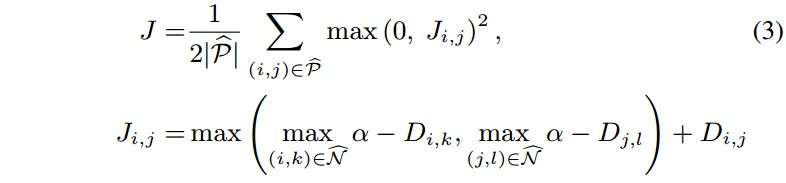${ \check{P} }$ - 训练集中的 positive 样本对 集合
${ \check{N} }$ - 训练集中的 negative 样本对 集合

• 非平滑

• 1 - 对函数的平滑上边界进行优化；
• 2 - 采用随机(stochastic)方法对大数据集处理.

• 1 - 偏向于对“困难”样本对，正如 $J_{i,j}$ 的 subgradient 计算 采用的接近的 negative pairs. (it biases the sample towards including “difficult” pairs, just like a subgradient of Ji;j would use the close negative pairs).
• 2 - 对一次采样的 mini-batch 内的所有样本信息进行利用，而不仅是单独的样本对.

key idea：

• 加速 mini-batch 的优化，以充分利用 batch 内的全部 $O(m^2)$ 对样本信息 .

${ D^2 = \check{\pmb{x}} \pmb{1}^T + \pmb{1} \check{\pmb{x}}^T- 2XX^T }$

${ D_{ij}^2 = ||f(\pmb{x}_i)- f(\pmb{x}_j)||_2^2 }$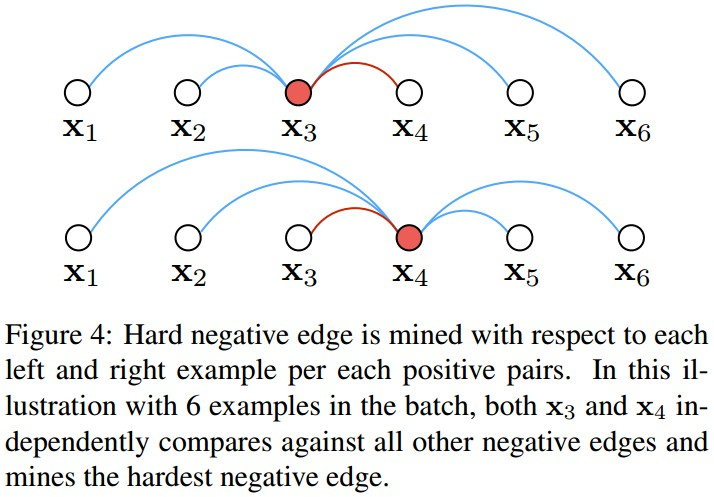Figure 4. 对于各 positive pairs的每个样本，找出其左右的 hard negative edge. batch内有 6 个样本，x3 和 x4 分别与所有的其它 negative edges进行比较，以找到 hardest negative edge.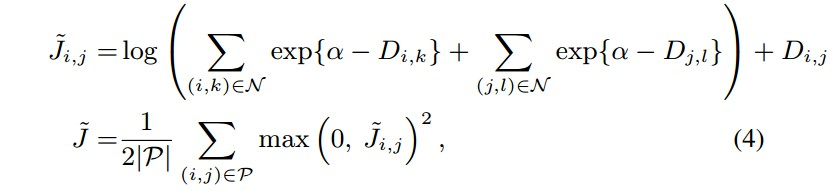BP计算过程：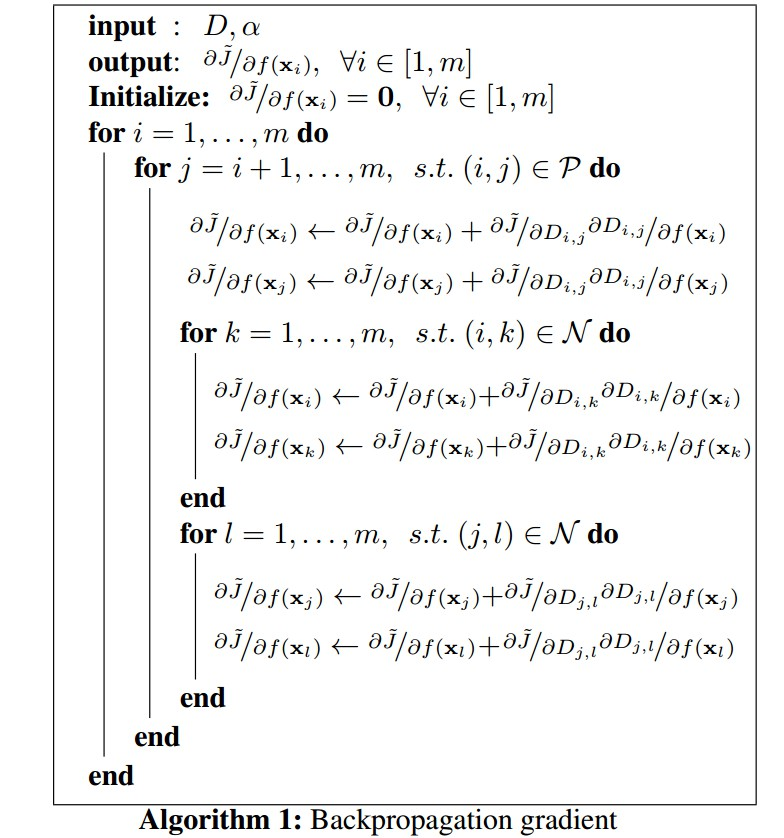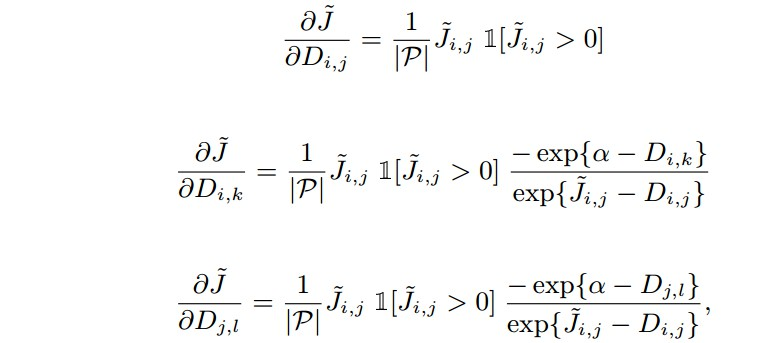## 3. Results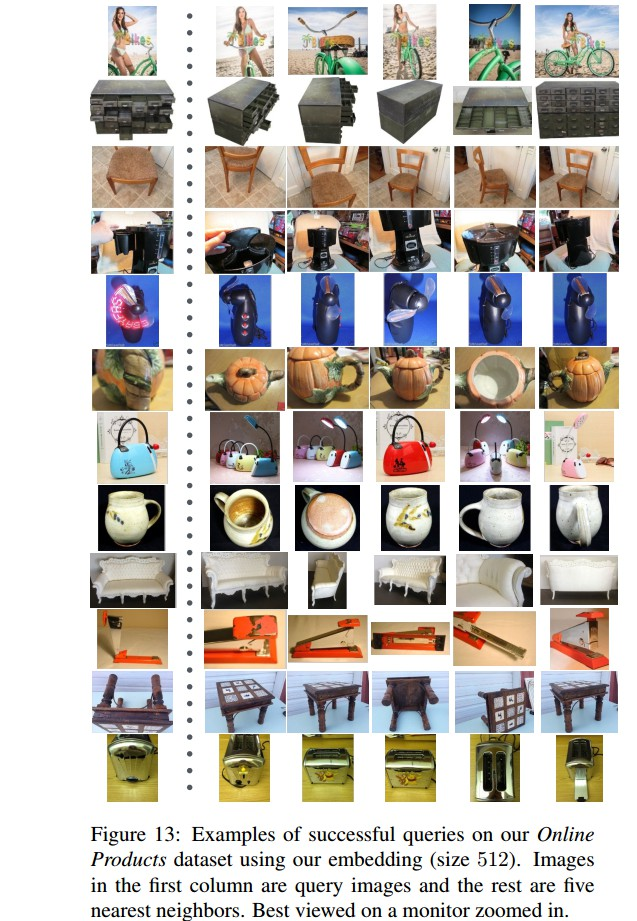## Reference

Last modification：February 4th, 2021 at 01:44 pm